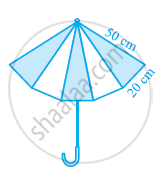# An umbrella is made by stitching 10 triangular pieces of cloth of two different colours (see the given figure), each piece measuring 20 cm, 50 cm and 50 cm. How much cloth of each colour is required for the umbrella? - Mathematics

An umbrella is made by stitching 10 triangular pieces of cloth of two different colours (see the given figure), each piece measuring 20 cm, 50 cm and 50 cm. How much cloth of each colour is required for the umbrella?#### Solution

For each triangular piece,

Semi-perimeter,

s=(20+50+50)/2=60 cm

By Heron’s formula,

"Area of triangle "=sqrt(s(s-a)(s-b)(s-c))

"Area of each triangle piece "=[sqrt(60(60-50)(60-50)(60-20))]cm^2

=[sqrt(60(10)(10)(40))]cm^2

=200sqrt6cm^2

Since there are 5 triangular pieces made of two different coloured cloths,

"Area of each cloth required "=(5xx200sqrt6)cm^2=1000sqrt6cm^2

Concept: Application of Heron’s Formula in Finding Areas of Quadrilaterals
Is there an error in this question or solution?
Chapter 12: Heron's Formula - Exercise 12.2 [Page 207]

#### APPEARS IN

NCERT Class 9 Maths
Chapter 12 Heron's Formula
Exercise 12.2 | Q 6 | Page 207

Share